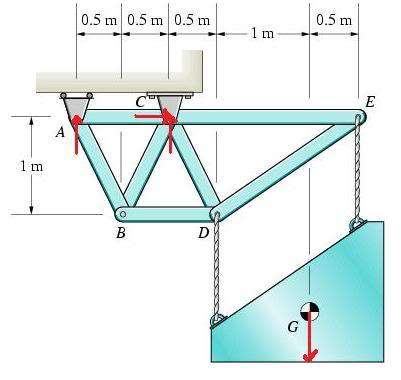# 2D Moment Equilibrium Question

## Homework Statement

A speaker system is suspended by the cables attached at and . The mass of the speaker system is 92.5kg, and its weight acts at G. Determine the tensions in the cables hanging from (a)D and (b)E .## Homework Equations

$$\sum$$M=0
$$\sum$$F=0

## The Attempt at a Solution

First, I decided to find reactions at C. I drew the arrows on the diagram, where the horizontal one going to C is Cx and vertical one is Cy. Vertical arrow pointing to A will be further referred to as Ay.
So I got:
Mc=Mcdue to A+Mcdue to G=0
Mcdue to A=92.5*9.81*1.5
Ay * 1 = 1361.14N
Since the moment is positive, Ay has to be pointing downwards
Thus Ay (vector)=-1361.14N. From this, we can find Cy:
$$\sum$$Fy = -1361.14N+Cy-907.425N=0
so Cy=2269
There are no x-direction forces, so Cx=0

Now that we know reactions at C, we can "cut" the cables (E&D) and find E using moment around D:
MD=+(1.5)*(1361.14)-2269*(.5)-(1)(0) - E*1.5=0
From which E (vector)=-604.81

Then we can use E, to find D:
Sum of Fy = -1361.14+2269-604.81+D=0
D=-303.05

Is that right? Or have I made some wrong assumptions?
Also, I got negative numbers for E and D because they're forces that are acting down. But how would I put in the answer? Tension should be positive 'cause it's just a magnitude, right?
Thanks!

Last edited: